# Multiplication Worksheets Grade 4

Download Multiplication Worksheets Grade 4Q.1) 7 x 4 = ?
a) 28
b) 21
c) 14

Q.2) 9 x 8 = ?
a) 72
b) 63
c) 54

Q.3) 15 x 9 = ?
a) 120
b) 135
c) 150

Q.4) 14 x 8 = ?
a) 140
b) 126
c) 112

Q.5) Which of the following equations shows the Associative Property of Multiplication?
a) 14 x 6 = 6 x 14
b) 6 x 1 = 6
c) ( 14 x 6 ) x 18 = 14 x ( 6 x 18 )

Q.6) Which of the following equations shows the Commutative Property of Multiplication?
a) ( 20 x 12 ) x 8 = 20 x ( 12 x 8 )
b) 12 x 20 = 20 x 12
c) 20 x 1 = 20

Q.7) Find the product :
14 x 200 = ?
a) 2800
b) 280
c) 1400

Q.8) Find the product.
5346 x 3 = ?
a) 15620
b) 15754
c) 16038

Q.9) Find the product.
324 x 25
a) 8100
b) 7920
c) 8420

Q.1) Explanation – Multiplication Worksheets Grade 4

According to the Table of 7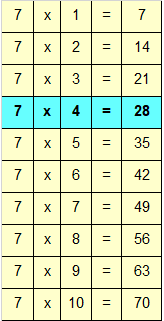If we look at the Table, we would get 7 x 4 = 28

Correct Answer – a) 28

Q.2) Explanation -Multiplication Worksheets Grade 4

According to the Table of 9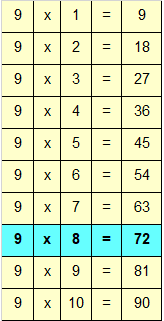If we look at the Table, we would get 9 x 8 = 72

Correct Answer – a) 72

Q.3) Explanation – Multiplication Worksheets Grade 4

According to the Table of 15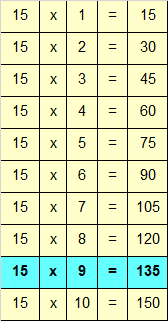If we look at the Table, we would get 15 x 9 = 135

Correct Answer – b) 135

Q.4) Explanation – Multiplication Worksheets Grade 4

According to the Table of 14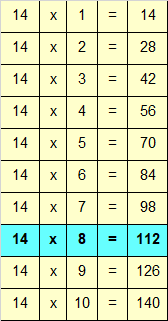If we look at the Table, we would get 14 x 8 = 112

Correct Answer – c) 112

Q.5) Explanation – Multiplication Worksheets Grade 4

According to the Associative Property of Multiplication, if we multiply more than two numbers, they can be grouped but the product remains the same i.e,

(a x b) x c = a x (b x c)

Hence, ( 14 x 6 ) x 18 = 14 x ( 6 x 18 ) satisfies Associative Property of Multiplication.

Correct Answer – c) ( 14 x 6 ) x 18 = 14 x ( 6 x 18 )

Q.6) Explanation – Multiplication Worksheets Grade 4

According to the Commutative Property of Multiplication, if we change the order of Multiplicand and Multiplier, the product remains the same i.e,

a x b = b x a

Hence, 12 x 20 = 20 x 12 satisfies Commutative Property of Multiplication.

Correct Answer – b) 12 x 20 = 20 x 12

Q.7) Explanation – Multiplication Worksheets Grade 4

To Multiply a given number with a Multiplier having zero at the end, we first count the number of zeros.

In 200 there are 2 zeros

Now we would multiply, the remaining digits of the given numbers, excluding zeros

i.e

Multiplying 14 by 2

14 x 2 = 28

Add 2 zeros after the product 28

The number would be 2800

Hence

14 x 200 = 2800

Correct Answer – a) 2800

Q.8) Explanation – Multiplication Worksheets Grade 4

Step I: Multiply 6 in ones place with 3
6 x 3 = 18

Since, the number is more than 9, we need to carry over 1 to the tens place.

Write 8 in ones place.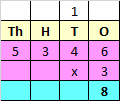Step II: Multiply 4 in tens place with 3
4 x 3 = 12
Add the carry over of 1 to 12
12 + 1 = 13
Since, the number is more than 9, we need to carry over 1 to the hundreds place.
Write 3 in tens place.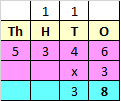Step III: Multiply 3 in hundreds place with 3
3 x 3 = 9
Add the carry over of 1 to 9
9 + 1 = 10
Since, the number is more than 9, we need to carry over 1 to the thousands place.
Write 0 in hundreds place.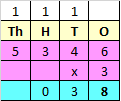Step IV: Multiply 5 in thousands place with 3
5 x 3 = 15
Add the carry over of 1 to 15
15 + 1 = 16
Since, the number is more than 9, we need to carry over 1 to the ten thousands place.

Write 1 in ten thousands place and 6 in thousands place.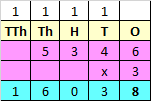Hence, 5346 x 3 = 16038

Correct Answer – c) 16038

Q.9) Explanation – Multiplication Worksheets Grade 4

Whenever we multiply a three digit Multiplicand with two digit Multiplier, we first multiply the Multiplicand with ones place of Multiplier, and then multiply Multiplicand with Tens place of the Multiplier.

Step I : Multiply Multiplicand with ones place of Multiplier.

i.e, 324 x 5 = 1620​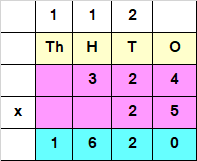Step II : Multiply Multiplicand with tens place of Multiplier.

i.e, 324 x 2 = 648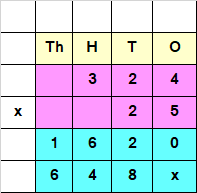Since the Number obtained at Step II, has a Tens value, we add a “0” or “x” at the end of the answer obtained by multiplying Multiplicand with Tens place of the Multiplier.

Step III : Add both the numbers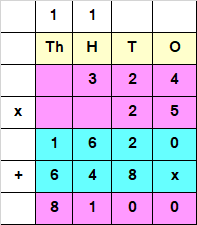Hence, 324 x 25 = 8100

Correct Answer – a) 8100